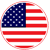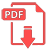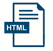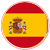# Home | Mathematics | Pre-Algebra

## Difference of Two Cubes

The other two special factoring formulas you'll need to memorize are very similar to one another; they're the formulas for factoring the sums and the differences of cubes. Here are the two formulas:

Factoring a Sum of Cubes:

a3 + b3 = (a + b)(a2 – ab + b2)

Factoring a Difference of Cubes:

a3 – b3 = (a – b)(a2 + ab + b2)

You'll learn in more advanced classes how they came up with these formulas. For now, just memorize them.

To help with the memorization, first notice that the terms in each of the two factorization formulas are exactly the same. Then notice that each formula has only one "minus" sign. The distinction between the two formulas is in the location of that one "minus" sign:

For the difference of cubes, the "minus" sign goes in the linear factor, a – b; for the sum of cubes, the "minus" sign goes in the quadratic factor:
a2– ab + b2.EnglishSpanish